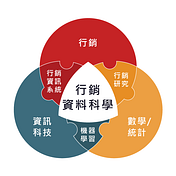# 一、事前檢驗

`import scipy.stats as stst.shapiro(alist)st.shapiro(blist)st.shapiro(clist)`
`ShapiroResult(statistic=0.9915865659713745,pvalue=0.6819638013839722)ShapiroResult(statistic=0.9929247498512268,pvalue=0.805463969707489)ShapiroResult(statistic=0.9802003502845764,pvalue=0.07433854043483734)`
`st.levene(alist, blist, clist, center='mean')`
`LeveneResult(statistic=0.6891243668404422,pvalue=0.5026819827621525)`

# 二、變異數分析

`f_value, p_value = st.f_oneway(alist, blist, clist)p_value`
`0.0955577857122512`

# 三、事後檢定

`from pingouin import pairwise_tukeym_comp = pairwise_tukey(data=data, dv='消費金額', between='廣告')`
`table = m_comp.drop(columns = ['mean(A)', 'mean(B)', 'T', 'p-tukey', 'hedges'])`

# 四、進階資料處理

`# 「A」欄反轉資料add_A = table['B'].tolist()# 「B」欄反轉資料add_B = table['A'].tolist()# 「diff」欄反轉資料diff =  (table['diff'] - 2 * table['diff']).tolist()# 「se」欄反轉資料se = table['se'].tolist()# 將反轉資料合併table2 = pd.DataFrame(zip(add_A, add_B, diff, se), columns = ['A', 'B', 'diff', 'se'])`
`new_table = pd.concat([table, table2], ignore_index=True)`
`new_table['上界'] = new_table['diff'] + new_table['se']*1.96new_table['下界'] = new_table['diff'] - new_table['se']*1.96`
`import numpy as npnp.sign(12)np.sign(-5.6)`
`1-1.0`
`justice = []for i in range(0,new_table.shape):      a = np.sign(new_table.iloc[i,4]) # 上界正負數判斷      b = np.sign(new_table.iloc[i,5]) # 下界正負數判斷      if a == b:          justice.append('Yes')      else:         justice.append('No')`
`new_table['是否顯著'] = justice`

# 五、資料視覺化

`import plotly.offline as pyimport plotly.graph_objects as gofig = go.Figure()  for i in range(0, new_table.shape):      if new_table.iloc[i,6] == 'Yes':          color = 'firebrick'          name = '顯著'      else:          color = 'green'          name = '不顯著'      fig.add_trace(go.Scatter(          x = [new_table.iloc[i,5], new_table.iloc[i,2], new_table.iloc[i,4]],          y = [new_table.iloc[i,0] + '-' + new_table.iloc[i,1], new_table.iloc[i,0] + '-' + new_table.iloc[i,1], new_table.iloc[i,0] + '-' + new_table.iloc[i,1]],          mode = "lines+markers",          textfont=dict(family="sans serif",              size=16,              color=color),                line=dict(color=color, width=2),          name = name,          legendgroup = name,          ))`
`fig.update_layout(      title={          'text': "<b>One-Way ANOVA 廣告效益分析</b>",          'y':0.95,          'x':0.5,          'xanchor': 'center',},      width=1800,      height=960,      boxmode='group',      font=dict(          family="Courier New, monospace",          size=20,          color="lightslategrey"      )      )`
`# 另存互動式網頁py.plot(fig, filename='One-Way ANOVA 廣告效益分析', auto_open=True)# 另存.png圖檔fig.write_image("C:/Users/user/Desktop/單因子變異數分析1.5/One-Way ANOVA 廣告效益分析.png")`
1. 平均消費金額在不同廣告推播下的差異區間，紅線代表顯著有差異，綠色代表顯著無差異。
2. 而圖中唯一有顯著差異的組合為廣告1以及廣告2，可看出廣告1帶來的平均消費金額顯著地大於廣告2。

--

--

--

## More from 行銷資料科學

Love podcasts or audiobooks? Learn on the go with our new app.## The Sibling Effect Ⅱ- Woodmam## Omniverse — A brief view